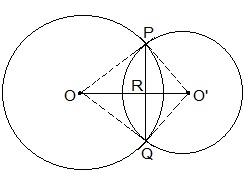Guru

# If two circles intersect at two points, prove that their centres lie on the perpendicular bisector of the common chord. Q.3

• 0

I want to know the best answer of the question from Circles chapter of class 9th ncert math. The question from exercise 10.3 of math. Give me the easy way for solving this question of 3  If two circles intersect at two points, prove that their centers lie on the perpendicular bisector of the common chord.

Share

1.It is given that two circles intersect each other at P and Q.

To prove:

OO’ is perpendicular bisector of PQ.

Proof:

Triangle ΔPOO’ and ΔQOO’ are similar by SSS congruency since

OP = OQ and O’P = OQ (Since they are also the radii)

OO’ = OO’ (It is the common side)

So, It can be said that ΔPOO’ ΔQOO’

∴ POO’ = QOO’ — (i)

Even triangles ΔPOR and ΔQOR are similar by SAS congruency as

POR = QOR (As POO’ = QOO’)

OR = OR (Common arm)

So, ΔPOR ΔQOR

∴ PRO = QRO

Also, we know that

PRO+QRO = 180°

Hence, PRO = QRO = 180°/2 = 90°

So, OO’ is the perpendicular bisector of PQ.

• 0